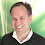## February 21, 2016

### Introduction

A very puzzling thought has been on my mind for a while now: is it possible to create a mechanical puzzle, something like the Rubik's Cube, that is unsolvable in general, but still demonstrably solvable if one knows the scrambling moves.

Such a puzzle would be truly mind-boggling and incredible frustrating for puzzle-solvers. Imaging a puzzle that starts from the solved state, just like a Rubik’s cube with all sides completed. Then, a long enough sequence of random moves (a scrambling sequence) would make it impossible to go back to the solved state if the scrambling sequence is not known. If the scrambling sequence is known, it is however trivial to reach the solved state by simply applying the inverse of each move in the scrambling sequence in reverse order.

### The definition of unsolvable

By impossible to solve or unsolvable, we mean that it is infeasible for a human to reach the solved state given a scrambled puzzle. To be concrete: there should be no general algorithm that solves the puzzle in less than 100 million moves. This roughly corresponds to one move every second for three years. Of course, one could always be lucky and find the solution faster, but on average, there should be no way to solve the puzzle in less than 100 million moves. And this is by my definition "infeasible" for a human to do.

### Notation and puzzle properties

Let's go ahead and define this problem more rigorously.

Consider a mechanical puzzle with a finite distinct number of states. From a particular puzzle state, S, another state can be reached by making a move. A move, m, is a mathematical function from one puzzle state to the next.

Si = mi(S_i-1)

OK, that looks ugly. MathML looks much better:

but it is slow to edit and does not work on all browsers (mobile phone browsers in particular). Let's go on using the ugly style.

Every move, m, has a corresponding inverse move, m'. So, for every move, m, and every state, S, there is an inverse move, m', such that:

S = m'(m(S))

When dealing with long move sequences, the function notation with all the resulting parenthesis is too annoying, instead we will use the following notation:

S3 = S0 | m1 m2 m3

to mean that when moves m1, m2, and m3 are applied to state S0, the resulting state is S3. So, with the new notation, we have:

Si = Si-1 | mi

and

S = S | m m'

Each puzzle state has a corresponding finite set of possible next moves. In the case of the Rubik's Cube, each state has 12 possible next moves (only 90 degree moves are considered, "quarter-turn metrics"). Often they are denoted: F, Fi, B, Bi, U, Ui, D, Di, L, Li, R, Ri.

The puzzle has one particular state called the solved state. Solving the puzzle is equivalent to finding a move sequence that transforms the current state to the solved state.

A scrambling of the puzzle is a random sequence of moves starting from the solved state. At each state, the next move is chosen randomly. Each possible next-move has the same probability of being chosen. A random scrambling of size n, is a random scrambling with n moves.

Now we are ready to summarize the properties of our puzzle. Lets call it an F-puzzle to distinguish it from other puzzles. An F-puzzle is a puzzle with the following properties:
• The puzzle has a finite number of states.
• One puzzle state is called the solved state.
• Each puzzle state has a set of next possible moves. The set is finite and has a size of at least two.
• For any state, S, and any move, m, there is an inverse move, m', such that:
S = S | m m'.
• A scrambling is a random sequence of moves starting from the solved state. The state after a scramble of size N is:
S_scrambled = S_solved | m1 m2 m3 ... mN.
• A scrambling is trivial to unscramble given that the scramble sequence is known:
S_solved = S_solved | m1 m2 m3 ... mN mN' m(N-1)' ... m3' m2' m1'
Hopefully, that is precise enough. Tell me if the F-puzzle definition is not clear.

The Rubik’s Cube is one example of an F-puzzle. However, it is solvable. In particular, any scrambling of the Rubik’s Cube can be solved in 20 moves in half-turn metrics and 26 moves in quarter-turn metrics. See www.cube20.org.

### The problem

So, now we can state the problem.

1. Can we create a mathematical unsolvable F-puzzle?

2. Can we create a mechanical unsolvable F-puzzle?

3. Can we create a practical mechanical unsolvable F-puzzle that can be mass-produced with a unit cost below 100 Euro?

To be concrete, we would like a mechanical puzzle that cannot be solved in 100 million moves after a scrambling of size 100 or less (20 or less would be nice). By "practical", we mean a puzzle that can be scrambled or unscrambled within one minute by most humans without training or tools. We also want a solution that is not a "mechanical computer"; it should be more of a Rubik's cube than a mechanical computer where symbols are used. For example, we don't want something like a code lock where digits are used to set a code.

That's the problem. Let's discuss potential solutions some other time.

1.The doctoral dissertation "Physical One-Way Functions" by Pappu Srinivasa Ravikanth may be relevant, not sure, did not read it yet.

2.Did you read it yet? :) I just found that dissertation and was looking for Ravikanth, which led me to your site.

3.I just skimmed through it, not sure if he has the answer. Perhaps...

4.